Electron. J. Diff. Eqns., Vol. 1994(1994), No. 04, pp. 1-10.

### Existence results for non-autonomous elliptic boundary value problems V. Anuradha, S. Dickens, & R. Shivaji

Abstract:
We study solutions to the boundary value problems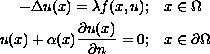where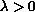,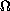is a bounded region in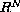;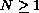with smooth boundary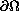,, n is the outward unit normal, and f is a smooth function such that it has either sublinear or restricted linear growth in u at infinity, uniformly in x. We also consider f such that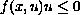uniformly in x, when |u| is large. Without requiring any sign condition on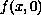, thus allowing for both positone as well as semipositone structure, we discuss the existence of at least three solutions for given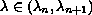where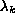is the k-th eigenvalue of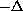subject to the above boundary conditions. In particular, one of the solutions we obtain has non-zero positive part, while another has non-zero negative part. We also discuss the existence of three solutions where one of them is positive, while another is negative, for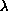near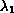, and forlarge when f is sublinear. We use the method of sub-super solutions to establish our existence results. We further discuss non-existence results forsmall.

Submitted January 23, 1994. Published July 8, 1994.
Math Subject Classification: 35J65.
Key Words: Elliptic boundary value problems, semipositone.

Show me the PDF(154K), TEX file, and other files for this article.

 V. Anuradha Department of Mathematics and Statistics University of Arkansas at Little Rock Little Rock, AR 72204-1099, USA S. Dickens Department of Mathematics and Statistics Mississippi State University Mississippi State, MS 39762, USAR. Shivaji Department of Mathematics and Statistics Mississippi State University Mississippi State, MS 39762, USA e-mail: shivaji@math.msstate.edu